# Transition to Chaotic Natural Convection of Cu-water Nanofluid in an Inclined Square Enclosure

Transition to Chaotic Natural Convection of Cu-water Nanofluid in an Inclined Square Enclosure

Bilal Boudjeniba | Abdelghani Laouer | Salah LaouarEl Hacene Mezaache

Département de Physique, Faculté des Sciences, Université de Skikda, B.P. 26, 21000, Algérie

Département de Physique, Université Mohamed seddikBenYahia - Jijel, B.P.98, Jijel 18000, Algérie

Laboratoire de Recherche en Physico-chimie des Surfaces et Interfaces, Université de Skikda, B.P.26, 21000, Algérie

Corresponding Author Email:
slaouar21@gmail.com
Page:
413-422
|
DOI:
https://doi.org/10.18280/ijht.370206
2 March 2019
|
Accepted:
4 June 2019
|
Published:
30 June 2019
| Citation

OPEN ACCESS

Abstract:

In this paper, the scenario leading to chaos in natural convection of Cu-water nanofluid inside an inclined square enclosure with the aspect ratio equal to unity is numerically investigated. The enclosure is heated from one part of the side and cooled through two other opposite half sides. The governing equations and the corresponding boundary conditions are solved numerically using the finite difference method. The effect of Rayleigh number and the volume fraction on natural convection flow are analyzed. The obtained results indicate that the mode of fluid flow which is initially stationary, passes by a periodic mode across a supercritical Hopf bifurcation, then quasi periodic at two incommensurable frequencies, before reaching the final stage of chaotic convection for both pure fluid and nanofluid. The sequences of bifurcation are presented graphically; it was found that the presence of suspended nanoparticles inside the base fluid causes significantly the delay of this transition.

Keywords:

heat transfer, natural convection, nanofluid, enclosure, Hopf Bifurcation, phase trajectory, Lyapunov exponent, chaos

1. Introduction

Transient natural convection inside the closed enclosures containing pure fluids has many practical engineering applications, the cases of electronic components cooling and especially the heat exchangers are among the most referenced applications. In all cases of the proposed topic, experimental studies and numerical computations aimed to clarify the flow regime and to determine the temperature field with both stable and instable boundary conditions. It should also to characterize the scenario leading to chaos and turbulence in relation with the nature of the fluid, the geometrical configuration of the enclosure and the initial and boundary conditions. In this context, we note the existence of some excellent research works related to this subject, which spans over sixty years. Nevertheless, the low thermal conductivity of fluids limits the performance of heat transfer inside enclosures. However, it should be noted that the presence of metal, metallic oxide or non-metallic nanometer-sized particles (referred to as MNS-particles, MONS-particles or NMNS-particles for convenience) in the fluid (named nanofluid firstly by Choi, 1995 ) contributes substantially to increase the heat transfer rate.

As far as we know, turbulent or chaotic natural convection in closed enclosures containing nanofluids has not been studied sufficiently, although this regime of flow seems to be very interesting. This implies that the solid particles neither settle at the bottom of a fluid nor in clustering.

Sadik et al.  summarize some important published works on the effect of MNS-particles, MONS-particles and NMNS-particles suspended in the base fluid on the forced convection heat transfer inside the enclosures. In the literature they include analytical models that agree with experimental data of several physical properties such as thermal conductivity, effective viscosity, density and effective heat capacity.

In the same way, Motevasel et al.  investigate experimentally at low concentration the effect of MgO-nanoparticles aggregation on the nanofluid’s thermal conductivity and viscosity and a relatively good agreements was found between the proposed fractal models and the experimental values.

Oztop et al.  considered rectangular enclosures partially heated and filled with nanofluid in natural convection. The finite volume method was used to solve the governing equations. For different types of nanoparticles: Cu, Al2O3 and TiO2, an increase in the mean Nusselt number was found with respect to the volume fraction andwith Rayleigh number inthe range of 1000-500000. Also, by using nanofluids, the heat transfer is more pronounced at low aspect ratio than at high ones, a value of 25 % can be reached.

Maiga et al.  studied numerical thermal forced convection of water/gAl2O3 and ethylene-glycol/gAl2O3 nanofluids inside cylindrical configuration with uniform heat flux at borders. For the turbulent flow, a Launder-Spalding k-e model was used with specific combined thermal and physical properties of the base fluids and nanoparticles. The solutions were validated by experimental data, it is noticed that the presence of particles affects the wall friction, this latter increases with the particle volume fraction.

In another study, Maiga et al.  considered two systems uniformly heated with Dirichelet and Neumann boundary conditions: the structure consists of a tube and parallel coaxial disks. They used two types of nanofluids in laminar forced convection flow regime, namely water/gAl2O3 and ethylene-glycol/gAl2O3. They noticed that this last nanofluid provides a better heat transfer. The presence of nanoparticles in the base fluid increases the wall shear stress substantially. The authors proposed two correlations for the averaged Nusselt number in relation with Reynolds number and Prandtl number to analyze the heat transfer in a tube using water as base fluid. In the same geometrical configuration of uniformly heated tube, Maiga et al.  explored numerically the effect of Al2O3 volume fraction on the hydrodynamic and thermal behavior of turbulent flow regime. The mathematical model based on the non-linear and coupled momentum of the energy and mass equations has been solved by a finite difference algorithm. The differential equations are discretized over a control volume. They supposed a single phase approach (e.g. fluid phase and particles in thermal equilibrium and move with the same velocity) and it was found that the wall shear stress increases with particle volume fraction and Reynolds number.

Elahmer et al. , on the effect of nanofluids in forced convective heat transfer in a tube, concludes thatethylene-glycol/CNT-Ag hybride nanofluid seems to be more advantageous for the enhancement of convective heat transfer than ethylene-glycol/CNT nanofluid.

The case of two-phase approach (each phase with his own velocity) has been used to study both forced and mixed convection of nanofluid in a tube [9-10] and in inclined square cavity . Some of the researchers consider that it is the appropriate approach to investigate in this novel kind of fluids. But there are some parameters which appear when using nanofluids, like gravity, Brownian motion, nanoparticles clustering and the friction between the fluid and the particles. Due to the lack of appropriate theoretical studies and experimental data in the literature to investigate in this issue, the existence of macroscopic two-phase approach is not applicable for analyzing heat transfer process [12-14]. Moreover, the single-phase approach is less complicated for modeling and more computationally efficient.

In order to maintain a nanofluid in homogeneous state and to maximize their capacity to evacuate heat, it is essential to maintain the nanofluid in the chaotic state. The aim of the present numerical study is to investigate on the different ways leading to chaos for Cu-water nanofluid in order to improve heat transfer by natural convection throughout closed enclosure and to avoid nanoparticles clustering and sedimentation. In this paper it is supposed that the fluid and the nanoparticles are in a single phase and homogeneous state. We consider an inclined closed square enclosure heated partially from one side and cooled at two opposite walls, while the rest of boundaries are adiabatic.

2. Mathematical Formulation

The geometry of the present study is an inclined enclosure filled with a Cu-water nanofluid, this is shown in Figure 1. It consists of an H and L as enclosure dimensions.

The half wall located at $x=0$ and the opposite one located at $x^{\prime}=L$ are maintained at the constant cold temperature $T_{c},$ whereas one part of wall located at $y^{\prime}=0$ is maintained at a constant hot temperature $T_{h},$ while the other borders of enclosure are adiabatic.

The nanofluid in the cavity is considered to be newtonian, it is incompressible and laminar with constant physical properties except the density variation due to the Boussinesq approximation. The thermo-physical properties of the base fluid and the Cu-nanoparticles are listed in Table 1. It is considered that the viscous dissipation, thermal radiation and the work due to the volume expansion are negligible.

## 1.png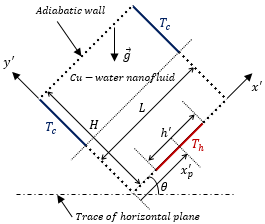Figure 1. Geometry of the problem and coordinate system.

Table 1. Thermophysical properties of the fluid and the nanoparticles, from 

 Physical properties Fluid phase (water) Copper (Cu) $c_{p}\left(J k g^{-1} K^{-1}\right)$ 4179 385 $\rho\left(k g . m^{-3}\right)$ 997.1 8933 $k\left(W . m^{-1} . K^{-1}\right)$ 0.613 400 $\alpha\left(m^{2} s^{-1}\right)$ $1.47 \times 10^{-7}$ $11.631 \times 10^{-5}$ $\beta\left(K^{-1}\right)$ $21 \times 10^{-5}$ $1.67 \times 10^{-5}$

Regarding the above assumptions, the dimensional governing equations using the vorticity stream-function formulation can be written in Cartesian coordinates as follows:

$\frac{\partial^{2} \psi^{\prime}}{\partial x_{l^{2}}}+\frac{\partial^{2} \psi^{\prime}}{\partial y^{\prime 2}}=-\omega^{\prime}$      (1)

$\frac{\partial \omega^{\prime}}{\partial t^{\prime}}+\frac{\partial}{\partial x^{\prime}}\left(u^{\prime} \omega^{\prime}-\frac{\mu_{n f}}{\rho_{n f}} \frac{\partial \omega^{\prime}}{\partial x^{\prime}}\right)+\frac{\partial}{\partial y^{\prime}}\left(v^{\prime} \omega^{\prime}-\frac{\mu_{n f}}{\rho_{n f}} \frac{\partial \omega^{\prime}}{\partial y^{\prime}}\right)$

$=\left(\frac{\varphi \rho_{s} \beta_{s}+(1-\varphi) \rho_{f} \beta_{f}}{\rho_{n f}}\right) g\left(\cos \theta \frac{\partial T^{\prime}}{\partial x^{\prime}}-\sin \theta \frac{\partial T^{\prime}}{\partial y^{\prime}}\right)$   (2)

$\frac{\partial T^{\prime}}{\partial t^{\prime}}+\frac{\partial}{\partial x}\left(u^{\prime} T^{\prime}-\alpha_{n f} \frac{\partial T^{\prime}}{\partial x \prime}\right)+\frac{\partial}{\partial y^{\prime}}\left(v^{\prime} T^{\prime}-\alpha_{n f} \frac{\partial T^{\prime}}{\partial y^{\prime}}\right)=0$  (3)

With the appropriate initial and boundary conditions:

For $t^{\prime}=0$ :

$\left\{\begin{array}{c}{u^{\prime}=v^{\prime}=0} \\ {T^{\prime}=\frac{1}{2}\left(T_{c}+T_{h}\right)}\end{array}\right.$  (4)

For $t^{\prime}>0$:

$\left\{\begin{array}{c}{u^{\prime}=v^{\prime}=0} \\ {\frac{\partial T^{\prime}}{\partial y^{\prime}}=0}\end{array}\right.$  at $\left[\begin{array}{c}{\left\{\begin{array}{c}{y^{\prime}=H} \\ {0 \leq x^{\prime} \leq L} \\ {y^{\prime} \leq 0} \\ {x^{\prime}<x_{p}^{\prime}-h^{\prime} / 2} \\ {x^{\prime}>x_{p}^{\prime}+\frac{h^{\prime}}{2}}\end{array}\right.}\end{array}\right.$

$\left\{\begin{array}{c}{u^{\prime}=v^{\prime}=0} \\ {\frac{\partial T^{\prime}}{\partial x^{\prime}}=0}\end{array} \text { at }\left\{\begin{array}{l}{x^{\prime}=0, x^{\prime}=L} \\ {0 \leq y^{\prime}<H / 2}\end{array}\right.\right.$

$\left\{\begin{array}{c}{u^{\prime}=v^{\prime}=0} \\ {T^{\prime}=T_{h}}\end{array} \text { at }\left\{\begin{array}{c}{y^{\prime}=0} \\ {x^{\prime}>x_{p}^{\prime}-\frac{h^{\prime}}{2}} \\ {x^{\prime}<x_{p}^{\prime}+\frac{h^{\prime}}{2}}\end{array}\right.\right.$

$\left\{\begin{array}{c}{u^{\prime}=v^{\prime}=0} \\ {T^{\prime}=T_{c}}\end{array} \text { at }\left\{\begin{array}{l}{x^{\prime}=0, x^{\prime}=L} \\ {H / 2 \leq y^{\prime} \leq H}\end{array}\right.\right.$ (5)

where

$\alpha_{n f}=\frac{k_{e f f}}{\left(\rho C_{p}\right)_{n f}}$   (6)

The effective thermal conductivity of the nanofluid $k_{e f f}$ is approximated by Maxwell-Garnetts model as cited in $[2,23],$ see Table 1 for calculations:

$\frac{k_{e f f}}{k_{f}}=\frac{k_{n f}}{k_{f}}=\frac{k_{s}+2 k_{f}-2 \varphi\left(k_{f^{-}}-k_{S}\right)}{k_{S}+2 k f^{+\varphi}\left(k_{f}-k_{S}\right)}$   (7)

We considered a spherical shape of copper nanoparticles [2, 4, 14, 22, 23]. This model is well adapted to evaluate the enhancement of the heat transfers. Other models exist in the literature, such as: the model of Hamilton-Crosser and the model of Yu-Choi, cited in , which takes into account the nonspherical form of the particles. For our case the use of the equation (7) is relatively satisfactory.

The specific heat of the nanofluid is expressed as [12, 15]:

$\left(\rho c_{p}\right)_{n f}=\varphi\left(\rho c_{p}\right)_{s}+(1-\varphi)\left(\rho c_{p}\right)_{f}$    (8)

The effective viscosity can be defined as follows :

$\mu_{n f}=\frac{\mu_{f}}{(1-\varphi)^{2.5}}$   (9)

Concerning the velocity field, it is related to the stream function by:

$\left\{\begin{array}{c}{u^{\prime}=\frac{\partial \psi^{\prime}}{\partial y^{\prime}}} \\ {v^{\prime}=-\frac{\partial \psi}{\partial x^{\prime}}}\end{array}\right.$   (10)

By introducing the following dimensionless variables:

$\left\{\begin{array}{c}{(x, y)=\left(\frac{x^{\prime}}{H}, \frac{y}{H}\right) ; t=\frac{t^{\prime} \alpha_{f}}{H^{2}} ;(u, v)=\left(\frac{u^{\prime} H}{\alpha_{f}}, \frac{v^{\prime} H}{\alpha_{f}}\right)} \\ {\psi=\frac{\psi^{\prime}}{\alpha_{f}} ; \omega=\frac{\omega^{\prime} H^{2}}{\alpha_{f}} ; \quad T=\frac{T^{\prime}-T_{c}}{T_{h}-T_{c}}}\end{array}\right.$  (11)

The governing equations (1)-(3) can be rewritten in dimensionless form as:

$\frac{\partial^{2} \psi}{\partial x^{2}}+\frac{\partial^{2} \psi}{\partial y^{2}}=-\omega$   (12)

$\frac{\partial \omega}{\partial t}+\frac{\partial}{\partial x}\left(u \omega-\operatorname{Prm} \frac{\partial \omega}{\partial x}\right)+\frac{\partial}{\partial y}\left(v \omega-\operatorname{Prm} \frac{\partial \omega}{\partial y}\right)=$

$R a . \operatorname{Pr} . \operatorname{Pnfr}\left(\cos \theta \frac{\partial T}{\partial x}-\sin \theta \frac{\partial T}{\partial y}\right)$   (13)

$\frac{\partial T}{\partial t}+\frac{\partial}{\partial x}\left(u T-t d r \frac{\partial T}{\partial x}\right)+\frac{\partial}{\partial y}\left(v T-t d r \frac{\partial T}{\partial y}\right)=0$   (14)

With the corresponding boundary conditions

For $t=0$:

$\left\{\begin{array}{c}{u=v=0} \\ {T=\frac{1}{2}}\end{array}\right.$    (15)

For  $t>0$:

$\left\{\begin{array}{c}{u=v=0} \\ {\frac{\partial T}{\partial y}=0}\end{array}\right.$   at $\left[\begin{array}{c}{\left\{\begin{array}{c}{y=1} \\ {0 \leq x \leq A} \\ {y=0}\end{array}\right.} \\ {\text {and}\left\{\begin{array}{c}{y=0} \\ {x>x_{p}-h / 2} \\ {x>x_{p}+\frac{h}{2}}\end{array}\right.}\end{array}\right.$

$\left\{\begin{array}{c}{u=v=0} \\ {\frac{\partial T}{\partial x}=0 \quad \text { at }\left\{\begin{array}{l}{x=0, x=A} \\ {0 \leq y<1 / 2}\end{array}\right.}\end{array}\right.$

$\left\{\begin{array}{c}{u=v=0} \\ {T=1}\end{array} a t\left\{\begin{array}{c}{y=0} \\ {x>x_{p}-\frac{h}{2}} \\ {x<x_{p}+\frac{h}{2}}\end{array}\right.\right.$

$\left\{\begin{array}{c}{u=v=0} \\ {T=0}\end{array} \text { at }\left\{\begin{array}{l}{x=0, x=A} \\ {1 / 2 \leq y \leq 1}\end{array}\right.\right.$ (16)

The dimensionless parameters appearing in the equations (13-14) are defined as

$t d r=\frac{\alpha_{n f}}{\alpha_{f}}=\frac{k_{n f}}{k_{f}} \frac{1}{(1-\varphi)+\varphi \frac{\left(\rho c_{p}\right)_{S}}{\left(\rho c_{p}\right)_{f}} )}$;

$\operatorname{Pr} m=\frac{\operatorname{Pr}}{(1-\varphi)^{2.5}\left((1-\varphi)+\varphi \frac{\rho_{S}}{\rho_{f}}\right)}$ ;

$R a=\frac{g \beta \Delta T L^{3}}{v_{f} \alpha_{f}}$;

$\operatorname{Pnfr}=\frac{1}{1+\frac{1-\varphi \rho_{f}}{\varphi \rho_{S}}} \frac{\beta_{s}}{\beta_{f}}+\frac{1}{1+\frac{\varphi \rho_{s}}{1-\varphi \rho_{f}}}$

For the estimation of the intensities of the heat transfers, the calculation of Nusselt number related to its local evaluation through the hot wall and its global evaluation along this wall. While referring to  and , it can be written as:

$N u_{l o c a l}(x)=-\frac{k_{n f}}{k f} \frac{\partial T}{\partial y} )_{h o t w a l l}$    (17)

Concerning the calculation of the averaged Nusselt number, this can be given as:

$N u_{A v g}=\int_{\text {hot wall}} N u(x) d x$   (18)

3. Numerical Method

The vorticity and energy equations are transformed into their finite difference equations by employing the ADI-method, which is adapted for the transient solutions. This formulation leads to a tri-diagonal matrix at each half time step that is solved using a TDMA algorithm. For this method, it is possible to use a relatively large time steps, in this case the numerical stability conditions are easy to satisfy.

To solve the stream function equation, the SOR-method is used. First of all, to satisfy the mass continuity, the convergence criterion of the stream function (e.g. the relative gap between the previous stream function at each point and their new values) is chosen less than 10-5. Secondly, the velocity components are computed with a central finite-difference approximation of the stream function. In the past, computational method has been tested for different mesh sizes (more than 121x121 nodes) and different time steps, thereafter, the validity of the computer program was tested for pure fluid in steady state by simulating the bench mark numerical solution . All results were considered to be very satisfactory (the relative gap less than 1 %). Other tests were carried out in oscillatory regimes and good agreement of frequencies is obtained. In addition, the results obtained by the present algorithm concerning the study of a nanofluid in a closed cavity with horizontal thermal walls are in good agreement with those presented by Oztop et al. .

4. Validation

To test the accuracy of the present numerical study, the average values of Nusselt number for wide range of Rayleigh numbers are given in Table 2 and compared with previous works. As it can be seen, the obtained results are in good agreements with those given by the literature.

Table 2. Comparison of average Nusselt number with those published in several references

 Ra Oztop  Khanafir  Davis  Tiwari  Esmaeil  Barakos  Present work 103 1.120 1.118 1.118 1.087 1.118 1.114 1.117 104 2.250 2.245 2.243 2.195 2.247 2.245 2.241 105 4.644 4.522 4.519 4.450 4.543 4.510 4.510 106 8.875 8.826 8.799 8.803 8.884 8.806 8.829

## 2.png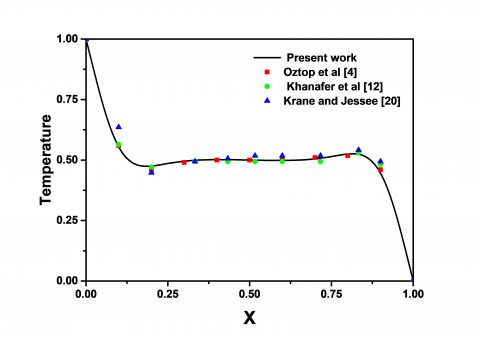Figure 2. Temperature profile at the horizontal mid-plane of the enclosure $R a=1.8910^{5}, P r=0.71$

## 3.pngFigure 3. Velocity profile at the horizontal mid-plane of the enclosure  $R a=10^{5}, h=0.5, y_{p}=0.5$

Another validation as shown in Figure 2, concerning natural convection in a square enclosure, differentially heated and filled with air. One can observe the obtained results of the computed temperatures, regarding the horizontal midline of the enclosure, comparison with the results of Oztop et al. , Khanafer et al.  and Krane et al.  gives excellent agreement.

The results obtained by simulation are validated with the result of Oztop et al.  for natural convection in a square enclosure filled with three types of nanoparticles showed in Figure 3. It is clear that the vertical velocity profile along the horizontal midline of the enclosure for Ra=105, h=0.5 and yp=0.5 is in good agreement with our numerical simulation.

As matter of comparison with Oztop et al. , a slight change in the volume fraction from zero to 0.20 causes a significant increase of average Nusselt numbers throughout a heated element as showed in Figure 4. Furthermore, it also considerable with increasing Rayleigh number. It is clear that all results of the present code (the one used for simulation) are in good agreement with those proposed by Oztop et al. .

## 4.png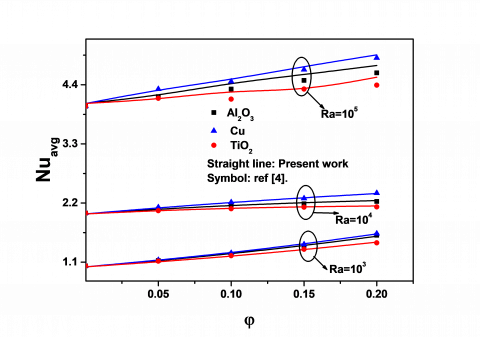Figure 4. Average Nusselt numbers versus volume fraction

5. Results and Discussion

The numerical code is used to make a number of simulations for various ranges of controlling parameter, such as Rayleigh number, $10^{3} \leq R a \leq 3.510^{7}$. The enclosure is partially heated at one side and partially cooled at two other opposite sides. Concerning the volume fraction, the used values are 10 % and 20 % in addition to the case of pure fluid.

5.1 Bifurcation to oscillatory convection

Figure 5, shows the temporal stream function signals at the mid-point of the enclosure (first line) in addition to the streamlines, the isotherms and the phase space trajectories at the second, third and fourth lines respectively. The first column refers to pure fluid $\left(R a=8.2010^{4}\right),$ the second column to Cu-water nanofluid with $\varphi=0.1\left(R a=1.9110^{5}\right)$ and the third column to Cu-water nanofluid with $\varphi=0.2$

$\left(R a=4.5210^{5}\right) .$ As indicated by this figure, the temporal signal shows a sharply decrease and then trend to an asymptotic limit of stationary heat transfer mode. We note that all physical properties of the system follow this evolution for studied cases.

## 5.png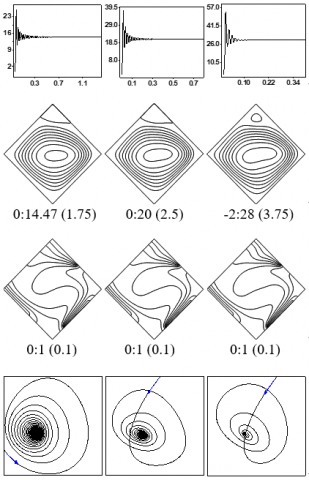Figure 5. Temporal stream function signals $\psi_{m i d}$  (line 1), streamlines (line 2), isotherms (line 3) and phase space trajectories $\left(T_{m i d}, \psi_{m i d}\right)$  (line 4).

For streamlines, the first number gives the minimum value, the second one, the maximum value and the third one is the gap between two successive streamlines.

The isotherms are equally spaced from the hot wall (T=1) to cold one (T=0), with a gap of 0.1

According to the streamlines, one can observe a dominate monocellular flow turning in the counterclockwise direction for all cases, whereas, a small counter rotating cell appears at the top corner of the enclosure, which grow up proportional with volume fraction. The corresponding isotherms show the characteristics of conduction for dominated regime except at the central part of the cavity, because they are distributed approximately parallel to the active walls. The stationarity of the regime can be confirmed through the layout of the phase plan trajectory ( $\left(T_{m i d}, \psi_{m i d}\right)$  for the threecases. Line four of Figure 5 shows that this is a limit point attractor.

When increasing Rayleigh number until obtaining an oscillatory convection mode, before reaching this situation precisely, we expect to find the breaking value of Rayleigh number, where there is a complete alteration of stationary regime behavior. We highlight that despite of increasing the Rayleigh number, the frequency of the cycle deduced by the Fourier spectrum remains almost invariant and the time required for the establishment mode becomes much longer near this value.

## 6.pngFigure 6. Temporal signals of  $\psi_{m i d}$  (first line), Phase space trajectories $\left(T_{m i d}, \psi_{m i d}\right)$  (second line), Amplitude spectra (third line) and the amplitude of $\psi_{\text { mid }}$  versus $\left(R a-R a_{c}\right)^{1 / 2}$  beside the bifurcation point (fourth line), respectively for Pure fluid (first column; Ra=8.40 104), nanofluid with φ=10 % (second column; Ra=1.97 105) and nanofluid with φ=20 % (third column Ra=4.60 105)

## 7.png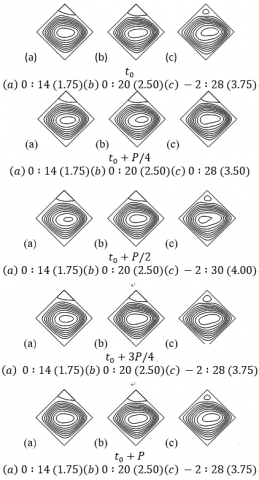Figure 7. Streamlines over one complete cycle: (a) Pure fluid at Ra=8.45 104; (b) Cu-water nanofluid with $\varphi=0.1$  at Ra=2.0 105; (c) Cu-water nanofluid with $\varphi=0.2$  at Ra=4.64 105

After several tests, we can determine with a good approach the value of Rayleigh number, at which there is a topological change of the temporal signals of stream function (first line, Figure 6) for several Rayleigh numbers, the signals become periodic. In the same way, sketch of phase space trajectories (second line, Figure 6) at the same values of Rayleigh numbers confirm the existence of a limiting cycle representing the phase portrait of stream function versus temperature at the mid-point of the cavity $\left(T_{\operatorname{mid}}, \psi_{\operatorname{mid}}\right)$. The third line illustrates the amplitude spectra with the non-dimensional frequencies values. Apparently, there is only one single frequency component for each case: $f=34.17, f=52.49$ and $f=0.1$ and $f=0.1$ and $f=0.1$ and Cu-water nanofluid with $\varphi=0.2$ respectively.

In the fourth line, from Figure 6, we show the amplitude of the stream function at the mid-point of the cavity for various Rayleigh numbers near the first critical point versus the square root differences between all used values of Rayleigh number and critical Rayleigh number for all cases studied here. Apparently, for the three cases, the evolution is linear, consequently, we deduce that there is a supercritical Hopf bifurcation $$ located near $R a=8.3510^{4}$ for pure fluid, $R a=1.9510^{5}$ for Cu-water nanofluid with $\varphi=0.1,$ and $R a=4.5610^{5}$ for Cu-water nanofluid with $\varphi=0.2$ . Note that these values were extracted by a linear fitting.

## 8.png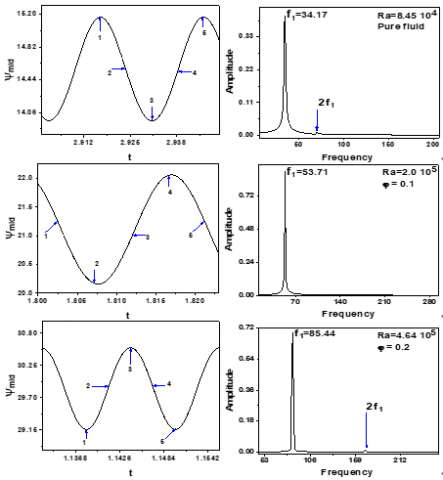Figure 8. Temporal signals of  $\psi_{m i d}$  (first column) and amplitude spectra (second column): First line: Pure fluid; Second line: Cu-water nanofluid with $\varphi=0.1$ ; Third line: Cu-water nanofluid with $\varphi=0.2$

Figure 7 shows streamlines over one complete cycle for pure fluid (a) $R a=8.4510^{4},$ Cu-water nanofluid (b) $R a=$ $210^{5} \varphi=0.1$ and Cu-water nanofluid (c) $R a=4.6410^{5} \varphi=$ 0.2 respectively at specific moments of the cycles (referring to 1-5, from Figure 8). One can observe mainly a single circulation cell turning in the counterclockwise direction in all cases and at the top corner of the enclosure one observes a small cell turning in the clockwise direction and growth at a specific moment of the cycle. In addition, Figure 8 shows that the flow is characterized by the presence of only one fundamental frequency with one small harmonic. Through these results, it is quite clear that the physical system has a periodic behavior.

5.2 Bifurcation to chaos

The bifurcation sequences are observed numerically until the onset of chaos, this is illustrated in Figure 9 (pure fluid), Figure 10 (Cu-water nanofluid with $\varphi=0.1$ ) and Figure 11 (Cu-water nanofluids with $\varphi=0.2$ ), where: the first column shows the time evolution of the temperature at the mid-point of the cavity, the second column presents the amplitude spectra of stream function, we note that the fast Fourier transform (FFT) algorithm is used to evaluate the amplitudes in the frequency domain, mostly 213 and 214 points, they were checked out from the temporal signals. Eventually, the third column shows the phase space trajectory in the plane $\left(T_{m i d}, \psi_{m i d}\right)$.

5.2.1 Bifurcation for pure fluid

According to Figure 9, the frequency amplitude spectrum for Ra=8.70 104 (first line) shows the non-dimensional fundamental peak frequency with order 35.4 and one significant harmonic of this peak is obvious. In addition, the phase space trajectory is a limit cycle, corresponding to a periodic state, related to the temporal signal of temperature at the mid-point of the cavity.

## 9.png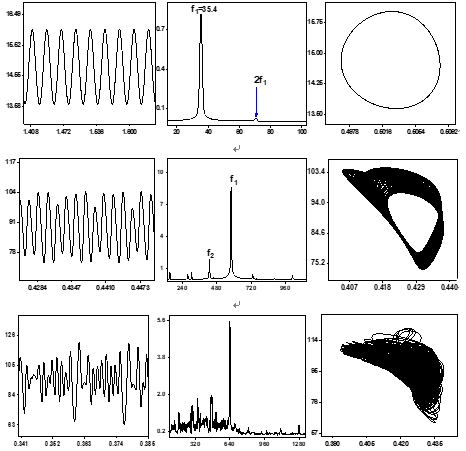Figure 9. Bifurcation sequence to chaos for pure fluid

As Rayleigh number increased further, we observe in the second line at Ra=8.25 106 that the amplitude spectrum resulting from FFT method includes a second frequency $f_{2}$ . It is noted that the ratio $f_{1} / f_{2}=1.354$  is irrational, where f1=578.61, f2=427.24; we define this situation as incommensurability, so the attractor is a T2 torus. As expected, this second bifurcation occurs for Rayleigh number ranging from 8,062,500 to 8,125,000.

The quasi periodic mode with two observable incommensurable frequencies is still existing for Ra=9.70 106 where f1=629.882, f2=463.867 and $f_{1} / f_{2} \cong 1.357$  and all the rest of peaks are: f1-f2, 2(f1-f2), 2f1, f1+f2. We note that the spectrum is highly noisy, which is precursor to pseudo-chaotic behavior.

The transition from quasi-periodic to chaotic flow can be clearly visualized in third line, where the Rayleigh number is 107, apparently the temporal signal of temperature has a non-periodic evolution, in addition the phase space trajectory is fully disturbed. We further note that the spectrum amplitude is continuous, which is similar to the characteristic exhibited by all chaotic regimes.

5.2.2 Bifurcation for Cu-water nanofluid

In this  section, we will explore the way towards the chaotic mode undergo by water containing copper nanoparticules with a volume fraction in the range [10-20 %], and see the influence of additional nanoparticles on the flow mode and we will try to compare  with the base case, i.e., water that is presented in the first part.

## 10.png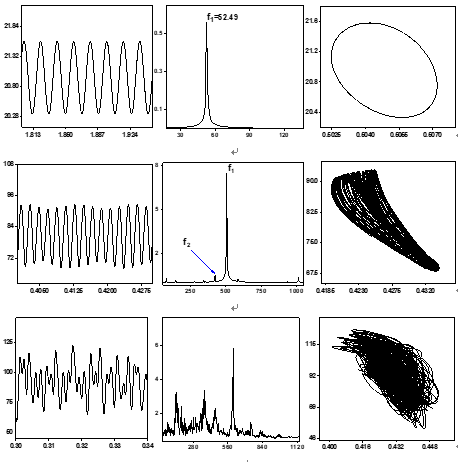Figure 10. Bifurcation sequence to chaos for Cu-water nanofluid withAccording to Figure 10, the frequency amplitude spectrum for Ra=1.97 105 (first line) shows the non-dimensional fundamental peak with order 52.49, since the temporal signal of temperature at the mid-point of the cavity have a periodic evolution, but no significant harmonic is obvious. In addition the phase space trajectory is a limit cycle, which is corresponding to the periodic state.

At Ra=106, we note also that the flow is strictly periodic containing one fundamental frequency. Similarly, the appearance of a single harmonic, has a frequency of a doubled value compared to the first one. As the Rayleigh number is increased to 1.109 107, we observe in the second line two distinct frequencies having irrational ratio, at which the periodic mode submits a second transition leading into a T2 torus. In this case harmonics are obtained from their linear combinations. In this region the flow is fully quasi-periodic as expected, since the second bifurcation occurs for Rayleigh number included in the interval [10,615,000; 11,090,000]. The quasi periodic mode with 2 observable incommensurable frequencies is still existing until Ra=1.35 107.

chaotic state occurs for Rayleigh number $R a>1.35$ 107, as an indication, Ra=1.40 107 in the last line. The amplitude spectrum has a continuous nature similar to the characteristic exhibited by all chaotic regimes. We note also, that the temperature signal is nonperiodic.

## 11.png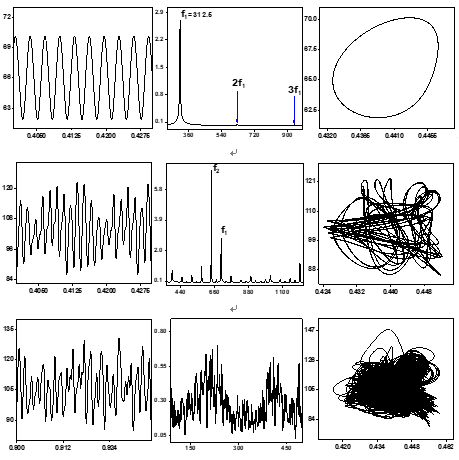Figure 11. Bifurcation sequence to chaos for Cu-water nanofluid with $\varphi=0.2$

From Ra number equal to 4.565 105 the regime is periodic, the dimensionless fundamental peak frequency is of the order of 84.22. At the first line, from Figure 11, Ra=5.0 106, it shows two harmonics in addition to fundamental one. The phase portrait shows a closed trajectory, corresponding to a periodic regime.

For Ra=2.60 107, by utilizing FFT method, we found in the second line from Figure 11 two distinct incommensurate frequencies: f1=700.68 and f2=637.20. In this case all harmonics can be expressed by linear combinations of these fundamental frequencies set as $m_{1} f_{1}+m_{2} f_{2}$ where $m_{1}$ and $m_{2}$ being positive integers. Consequently, the regime is quasi-periodic..

We observe in third line for Ra=2.80 107 that the temperature signal is non-periodic. The transition to chaotic flow can be clearly visualized through the phase space maps and from amplitude spectrum which has a continuous nature.

6. Sensitivity to the Initial Conditions

The sensitivity to the initial conditions of our system is highlighted following by a change of the initial temperature of the enclosure of the order of 10-6. Figure 12 gives the examples for various pertinent numbers of Rayleigh, (a): pure fluid, (b): Cu-water nanofluid with $\varphi=0.1$ , and (c): Cu-water nanofluid with $\varphi=0.2$ , where it is seen that the signals have the same shape until a given time, where the two signals become separate, this behavior is a sign of all chaotic systems.

## 12.png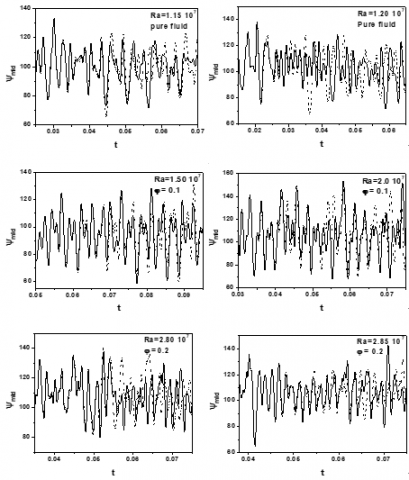Figure 12. Sensitivity to the initial conditions for: (a) pure fluid; (b) nanofluid with $\varphi=0.1$ ; (c) nanofluid with $\varphi=0.2$ ; Solid line, T01=0.5; dashed line, T02=0.5+10-6

The obtained results seem to be confirmed by the estimated largest Lyapunov exponent values Ly , where is defined by:

\begin{aligned}\left[\psi_{\operatorname{mid}}\left(T_{01}, t\right)-\psi_{\operatorname{mid}}\left(T_{02}, t\right)\right] & \\=&\left[\psi_{\operatorname{mid}}\left(T_{01}, 0\right)-\psi_{\operatorname{mid}}\left(T_{02}, 0\right)\right] \exp \left(L_{y} t\right) \end{aligned}  (19)

As it is shown in table 3, all values being positive and were proved to increase when Rayleigh number increases for both pure fluid and nanofluid. In the same way, calculations show that more increasing in Rayleigh number more the separation time occurs earlier.

Table 3. The estimated largest Lyapunov exponent values as a function of Rayleigh number

 Ra Lyapunov exponent $\varphi=0$ 1.15 107 170.3 1.20 107 177.72 1.22 107 245.16 $\varphi=0.1$ 1.40 107 75.47 1.50 107 124.59 2.0 107 184 $\varphi=0.2$ 2.80 107 162.21 2.85 107 171.07 3.10 107 244.82

Figures 13 summarize the study, in such way that, the transition from a regime of flow to another depends primarily on two parameters: Rayleigh number and volume fraction of the nanoparticules. Whereas, the addition of nanometric particle size to base fluid enables the slow downing of this transition in a proportional manner. So that, the attractor alters from limit point to a limit cycle via a supercritical Hopf bifurcation, and the values of Rayleigh numbers corresponding to this first bifurcation are 8.35 104, 1.95 105, 4.56 105 for the pure fluid, nanofluid with $\varphi=0.1$  and nanofluid with $\varphi=0.2$ , respectively. In the same way, the values of Rayleigh numbers agree with the two other transitions, i.e., transition from periodic to quasi periodic mode and quasi periodic to chaos for the three cases quoted previously.

## 13.pngFigure 13. Bifurcation sequences observed numerically. (a): pure fluid, (b): nanofluid with $\varphi=0.1$ , (c): nanofluid with $\varphi=0.2$ . S=steady state, P= periodic, QP2=quasi-periodic with two incommensurate frequencies, C= chaotic

According to Figure 13, we can also note that the quasi periodic regime exists in a narrow field of Rayleigh number, in such way, the chaotic mode is very close from this region.

7. Heat Transfer Improvement

## 14.png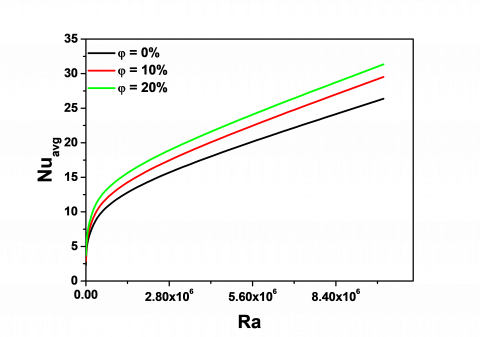Figure 14. Mean Nusselt number variation along the hot wall according to Rayleigh number and various volume fraction

In this section, the effect of volume fraction of copper nanoparticles on heat flow by natural convection in the cavity is studied, we have plotted various values of the mean Nusselt number along the hot wall for various volume fraction using different values of Rayleigh number as illustrated on Figure14. Since, the strength of natural convection is characterized by magnitude of a dimensional Nusselt number that compares a convective heat flow with reference to typical conductive heat flow. According to Figure 14, we notice that for three cases the heat transfer in the cavity is proportional to Rayleigh number. Let us indicate that the improvement of heat transfer is in relation with the volume fraction of copper nanoparticles, which is sustained by a wide range of authors [4, 12, 14]. The improvement of heat transfer when increasing volume fraction of nanoparticles is very evident. For example when Ra=106 and $\varphi=0 .1$ , the improvement is 10.39% compared to pure fluid, and it becomes 20.42% for $\varphi=0 .2$ .

8. Conclusion

The study proposed in this paper is carried out aiming to find out the physical instabilities for Cu-water nanofluid that occur in a square inclined cavity of  $45^{\circ}$, heated partially from one side and cooled partially from two other opposite side. From the investigation carried out in this paper, we were able to visualize the transition from steady state regime for low Rayleigh numbers until the onset of chaos when Ra increases.

The problem was treated using a numerical approach, based on the finite difference method and by using our own developed code, which is validated with experimental and numerical results, some of which are well explained in this study.

The scenario borrowed up to the chaos by the two systems (pure fluid and nanofluid) is according to that proposed by Curry and York , i.e., quasi-periodicity at two incommensurable frequencies. The transition from laminar natural convection to chaos is affected by the presence of nanoparticles, so that the volume fraction causes backwardness in the transition to chaotic regime, this is well explained from the diagram of Figure 13; but on the other hand an improvement in heat transfer inside the cavity was found.

A later study on unsteady natural convection in differentially heated cavities may be extended for other types of nanoparticles, taking into account other inclinations and various shape factors.

Acknowledgement

The authors would like to acknowledge Professor Mohamed Lashab from Larbi Ben M’hidi university, Algeria for his given assistance, we are extremely grateful to him for his help.

Nomenclature
 A geometric aspect ratio, L/H Cp specific heat at constant pressure, J.kg-1K-1 g acceleration due to gravity, m.s-2 Exp Exponential function h nondimensional length of partial heater, h’/L h’ length of heater, m H length of enclosure along y’, m k thermal conductivity, W.m-1.K-1 L length of enclosure along x’, m Ly Lyapunov exponent Nu Nusselt number P Period Pr Prandtl number t nondimensional time T nondimensional temperature Th hot wall temperature, K Tc cold wall temperature, K u, v nondimensional velocity components, x, y nondimensional cartesian coordinates xp Nondimensional position of the heater element along x yp Nondimensional position of the heater element along y Greek symbols α thermal diffusivity, m2.s-1 β coefficient of thermal expansion, K-1 θ inclination angle, rad μ dynamic viscosity, kg. m-1.s-1 ρ density, kg.m-3 φ nanoparticle volume fraction ψ nondimensional stream function ω nondimensional vorticity Exponent and subscripts ‘ for dimensional quantities Avg Average eff Effective f Fluid nf Nanofluid s Solid
References

 Choi, U.S., Eastman, J.A. (1995). Enhancing thermal conductivity of fluids with nanoparticles. ASME FED, FED, 231: 99-105.

 Kakaç, S., Pramuanjaroenkij, A. (2009). Review of convective heat transfer enhancement with nanofluids. International journal of Heat and Mass Transfer, 52(13): 3187-3196. https://doi:10.1016/j.ijheatmasstransfer.2009.02.006

 Motevasel, M., Nazar, A.R.S., Jamialahmadi, M. (2017). Experimental investigation of turbulent flow convection heat transfer of MgO/water nanofluid at low concentrations – Prediction of aggregation effect of nanoparticles. International Journal of Heat and Technology, 35(4): 755-764. https://doi.org/10.18280/ijht.350409

 Oztop, H.F., Abu-Nada, E. (2008). Numerical study of natural convection in partially heated rectangular enclosures filled with nanofluids. International Journal of Heat and Fluid Flow, 29(5): 1326-1336. http://doi.org/10.1016/j.ijheatfluidflow.2008.04.009

 Maiga, S.E., Nguyen, C.T., Galanis, N., Roy, G. (2004). Heat transfer behaviours of nanofluids in a uniformly heated tube. Superlattices Microstructure, 35(3-6): 543-557. https://doi.org/10.1016/j.spmi.2003.09.012

 Maiga, S.E., Palm, S.J., Nguyen, C.T., Roy, G., Galanis, N. (2005). Heat transfer enhancement by using nanofluids in forced convection flows. International Journal of Heat and Fluid Flow, 26(4): 530-546. http://dx.doi.org/10.1016/j.ijheatfluidflow.2005.02.004

 Maiga, S.E., Nguyen, C.T., Galanis, N., Roy, G., Maré, T., Coqueux, M. (2006). Heat transfer enhancement in turbulent tube flow using Al2O3 nanoparticle suspension. International Journal of Numerical Methods for Heat and Fluid Flow, 16(3): 275-292. https://doi.org/10.1108/09615530610649717

 Elahmer, M., Abboudi, S., Boukadida, N. (2017). Nanofluid effect on forced convective heat transfer inside a heated horizontal tube. International Journal of Heat and Technology, 35(4): 874-882. https://doi.org/10.18280/ijht.350424

 Mirmasoumi, S., Behzadmehr, A. (2008). Numerical study of laminar mixed convection of a nanoﬂuid in a horizontal tube using two-phase mixture model. Applied Thermal Engineering, 28(7): 717–727. https://doi.org/10.1016/j.applthermaleng.2007.06.019

 Behzadmehr, A., Saffar-Avval, M., Galanis, N. (2007).  Prediction of turbulent forced convection of a nanofluid in a tube with uniform heat flux using a two phase approach. International Journal of Heat and Fluid Flow 28(2): 211-219. https://doi.org/10.1016/j.ijheatfluidflow.2006.04.006

 Alinia, M., Ganji, D.D., Gorji-Bandpy, M. (2011). Numerical study of mixed convection in an inclined two sided lid driven cavity ﬁlled with nanoﬂuid using two-phase mixture model. International Communications in Heat and Mass Transfer, 38(10): 1428–1435. http://doi.org/10.1016/j.icheatmasstransfer.2011.08.003

 Khanafer, K., Vafai, K., Lightstone, M. (2003). Buoyancy-driven heat transfer enhancement in a two-dimensional enclosure utilizing nanofluids. International Journal of Heat and Mass Transfer, 46(19): 3639-3653. https://doi.org/10.1016/S0017-9310(03)00156-X

 Jou, R.Y., Tzeng, S.C. (2006). Numerical research on nature convective heat transfer enhancement filled with nanofluids in rectangular enclosures. Int. Commun. Heat Mass Transfer, 33(6): 727-736. http://doi.org/10.1016/j.icheatmasstransfer.2006.02.016

 Tiwari, R.K., Das, M.K. (2007). Heat transfer augmentation in a two sided lid-driven differentially heated square cavity utilizing nanofluids. International Journal of Heat and Mass Transfer, 50(9-10): 2002-2018. https://doi.org/10.1016/j.ijheatmasstransfer.2006.09.034

 Lee, S., Choi, S.U.S., Li, S., Eastman, J.A. (1999). Measuring thermal conductivity of fluids containing oxide nanoparticules. Trans. ASME, Journal Heat Transfer, 121(2): 280-289. http://doi:10.1115/1.2825978

 Boudjeniba, B., Laouar, S., Mezaache, E. (2015). Etude numérique de la convection naturelle dans une cavité rectangulaire contenant des nanofluides. Second International Conference on Machanics, Université Constantine1, pp. 25-26.

 De Vahl Davis, G. (1983). Natural convection of air in a square cavity: A bench mark numerical solution. International Journal for Numerical Methods in Fluids, 3(3): 249-264. https://doi.org/10.1002/fld.1650030305

 Esmaeil, K.K. (2013). Numerical feasibility study of utilizing nanofluids in laminar natural convection inside enclosures. Heat and Mass Transfer, 49(1): 41-54. https://doi.org/10.1007/s00231-012-1059-x

 Barakos, G., Mitsoulis, E. (1994). Natural convection flow in a square cavity revisited: laminar and turbulent models with wall functions. Int J Numer Methods Fluids, 18(7): 695-719. https://doi.org/10.1002/fld.1650180705

 Krane, R.J., Jessee, J. (1983). Some detailed field measurements for a natural convection flow in a vertical square enclosure. Proceedings of the First ASME-JSME Thermal Engineering Joint Conference, 1: 323-329.

 Gollub, J.P., Benson, S.V. (1980). Many routes to turbulent convection. J. Fluid Mech., 100(3): 449-470. https://doi.org/10.1017/S0022112080001243

 Palm, S., Roy, G., Nguyen, C.T. (2006). Heat transfer enhancement with the use of nanoﬂuids in a radial flow cooling systems considering temperature dependent properties. Appl. Therm. Eng., 26: 2209–2218. http://dx.doi.org/10.1016/j.applthermaleng.2006.03.014

 Gladés, B. (2010). Thèse de doctorat, Contribution à l’étude de la convection naturelle dans les nanofluides en configuration de Rayleigh-Bénard. Institut de mécanique des fluides de Toulouse (IMFT), UMR 5502, France.